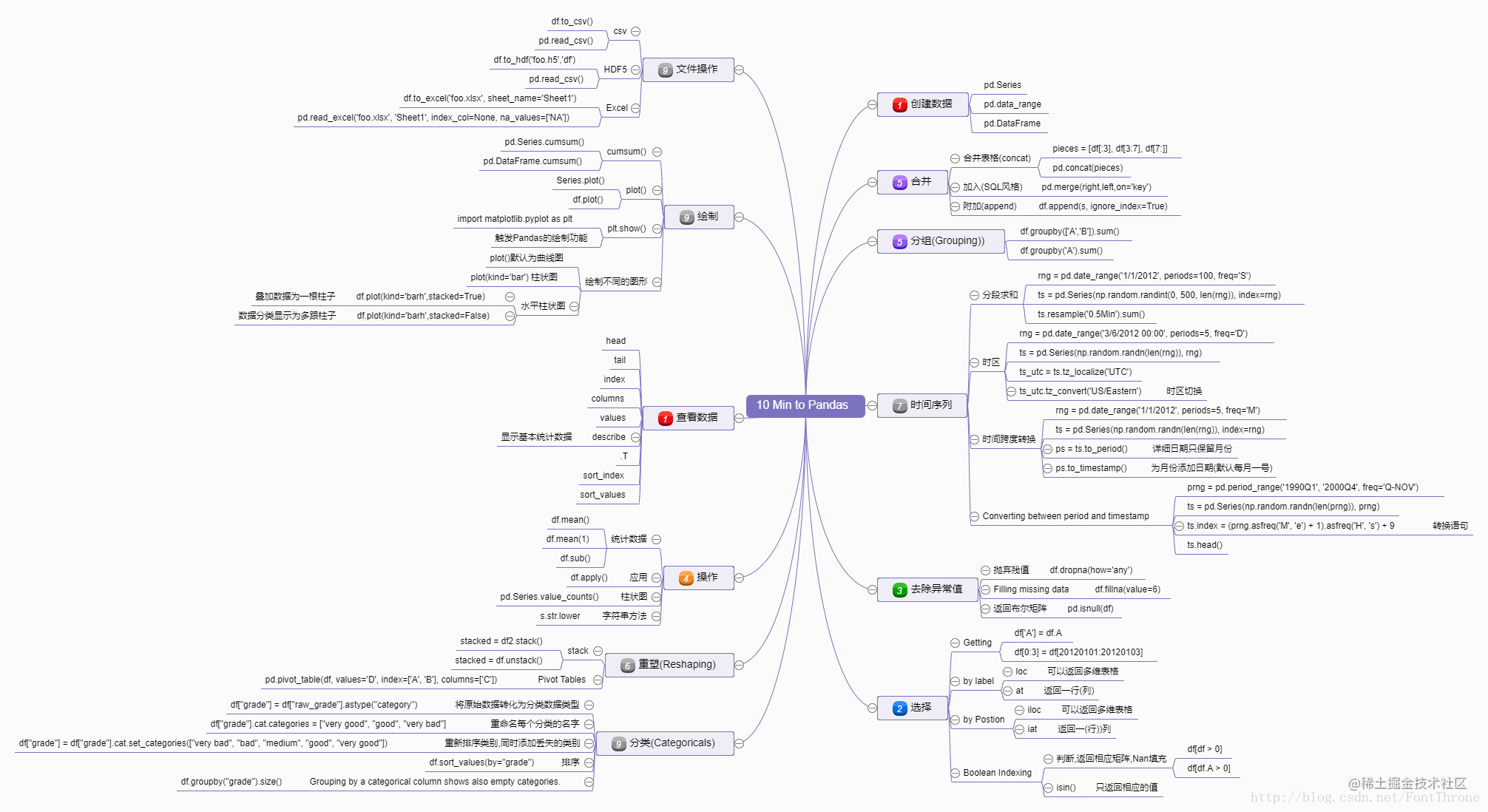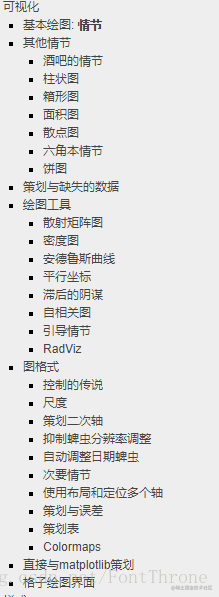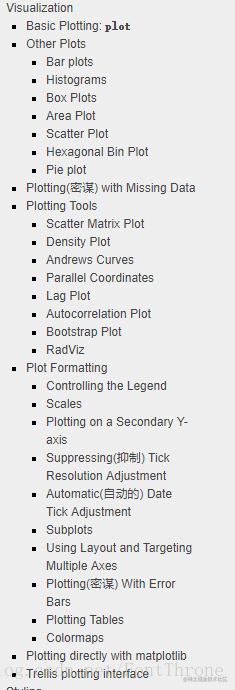# 如何快速掌握Pandas

## 如何快速入门

pandas是python的一个出色的统计类库,同时也是python的科学计算与可视化生态系统中不可或缺的一环,但是如此出色的pandas却十分的简单易上手而且拥有出色的官方文档,假如你想快速的掌握pandas的使用的话,那么官方文档必是首选.建议直接访问pandas官网:pandas.pydata.org/pandas-docs…,与有道网页翻译搭配食用效果更佳.

## 10 Minutes to pandas 快速入门## 要注意的几个地方

• 获取DataFrame长度
len(DataFrame.index)
• 文件读写问题:Unnamed
``````# 将DataFrame类型文件以csv格式导出的时候,再读入会产生一个新列'Unnamed: 0'
# 内容为DataFrame的index
# 有两种解决方案,第一手动删除

del df1['Unnamed: 0']
# 或在存储csv是不存储idnex,例如
# 不要列头，不要索引，只要open,high两列。来解决这个问题

• 文件读写问题:columns
• 更多文件读取问题
建议额外阅读官方文档pandas.pydata.org/pandas-docs…
• 画图问题:Pandas无法显示中文:
需要动态修改配置
``````import matplotlib as mpl
mpl.rcParams['font.sans-serif'] = ['KaiTi']
mpl.rcParams['font.serif'] = ['KaiTi']
# mpl.rcParams['axes.unicode_minus'] = False # 解决保存图像是负号'-'显示为方块的问题,或者转换负号为字符串

import seaborn as sns
sns.set_style("darkgrid",{"font.sans-serif":['KaiTi', 'Arial']})

• 添加更多的matplotlib 样式,这个只需要引入matplotlib 包然后使用plt添加即可,例如
``````import matplotlib.pyplot as plt
......

df= pd.DataFrame(listval, columns=['次数'])
df.index = listkey
df.plot(kind='bar')
plt.title('关于梅宏院士的报告的词频统计')

# 下面的这些代码都是有效的

# plt.figure(figsize = (8,4))
# plt.xlabel('x-变量',fontproperties=myfont,fontsize=12) #设置标签
# plt.ylabel('y-正弦余弦函数值',fontproperties=myfont,fontsize=12)
plt.show()

• pandas的绘图功能十分强大,但是要进行较为复杂的绘图,依旧建议使用matplotlib,pandas仅仅在统计绘图方面足够强大而已,好在使用numpy与pandas对接十分容易
更多的绘图功能建议详细阅读
官方文档目录: